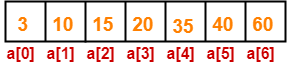## Searching Algorithms-

Before you go through this article, make sure that you have gone though the previous article on Searching Algorithms.

We have discussed that there are two approaches to searching-

1. Linear Search
2. Binary SearchIn this article, we will discuss about Binary Search.

## Binary Search-

• Binary Search is one of the fastest searching algorithms.
• It is used for finding the location of an element in a linear array.
• It works on the principle of divide and conquer technique.

## When Binary Search can be applied?

Binary search can be applied only on the sorted arrays where the elements are arranged in-

• Either ascending order if the elements are numbers
• Or dictionary order if the elements are strings

## Note-

To apply binary search on an unsorted array,

• First, sort the array using some sorting technique.
• Then, use binary search algorithm.

## How Binary Search Works?

Binary Search searches for an element by comparing it with the middle most element of the array.

Then, following three cases are possible-

### Case-01:

• If the element being searched is found to be the middle most element, its index is returned.

### Case-02:

• If the element being searched is found to be greater than the middle most element, then its search is further continued in the right sub array of the middle most element.

### Case-03:

• If the element being searched is found to be smaller than the middle most element, then its search is further continued in the left sub array of the middle most element.

This iteration keeps on repeating on the sub arrays until the desired element is found or size of the sub array reduces to zero.

## Binary Search Algorithm-

Consider-

• There is a linear array ‘a’ of size ‘n’ and binary search algorithm is being used to search an element ‘item’ in this linear array.
• If search ends in success, it sets loc to the index of the element otherwise it sets loc to -1.
• Variables beg and end keeps track of the index of the first and last element of the array or sub array in which the element is being searched at that instant.
• Variable mid keeps track of the index of the middle element of that array or sub array in which the element is being searched at that instant.

The algorithm is-

Begin

Set beg = 0

Set end = n-1

Set mid = (beg + end) / 2

while ( (beg <= end) and (a[mid] ≠ item) ) do

if (item < a[mid]) then

Set end = mid – 1

else

Set beg = mid + 1

endif

Set mid = (beg + end) / 2

endwhile

if (beg > end) then

Set loc = -1

else

Set loc = mid

endif

End

## Example of Binary Search-

Suppose element 15 is to be searched in the following array using binary search-The given array is already sorted in increasing order.

### Step-01:

To begin with, we take beg=0, end=6 and compute the location of the middle element as-

mid = (beg + end) / 2 = (0 + 6) / 2 = 3

Since a[mid] = a = 20 ≠ 15 and beg < end, we start next iteration.

### Step-02:

Since a[mid] = 20 > 15, so we take end = mid – 1 = 3 – 1 = 2 whereas beg remains unchanged.

Now, we compute the location of the middle element as-

mid = (beg + end) / 2 = (0 + 2) / 2 = 1

Since a[mid] = a = 10 ≠ 15 and beg < end, we start next iteration.

### Step-03:

Since a[mid] = 10 < 15, so we take beg = mid + 1 = 1 + 1 = 2 whereas end remains unchanged.

Now, we compute the location of the middle element as-

mid = (beg + end) / 2 = (2 + 2) / 2 = 2

Since a[mid] = a = 15 which matches to the element being searched, so our search terminates in success and index 2 is returned.

## Analysis of Binary Search-

• In each iteration or in each recursive call, the search is reduced to half of the array.
• So, for n elements in the array, there will be log2n iterations or recursive calls.

Thus,

#### Time Complexity

Time Complexity of Binary Search = O (log2n)

where n is the number of elements in the linear array

• This time complexity of binary search remains unchanged irrespective of the position of the element even if it is not present in the array.

## Advantages of Binary Search-

• It eliminates half of the list from further searching by using the result of each comparison.
• It indicates whether the element being searched is before or after the current position in the list and this information is used to narrow the search.
• For large lists of data, it works significantly better than linear search.

## Disadvantages of Binary Search-

• It employs recursive approach which requires more stack space.
• Programming binary search algorithm is error prone and difficult.
• The interaction of binary search with memory hierarchy i.e. caching is poor because of its random access nature.
• For in-memory searching, if the interval to be searched is small, linear search may exhibit better performance than binary search simply because it exhibits better locality of reference.

Get more notes and other study material of Design and Analysis of Algorithms (DAA).

Watch video lectures by visiting our YouTube channel LearnVidFun.